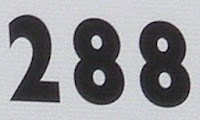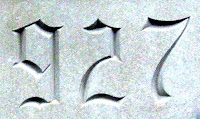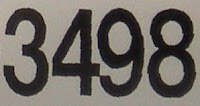## Tuesday, May 31, 2011

### 244

244 = 22 x 61.

244 has a representation as a sum of two squares: 244 = 102 + 122. It is the second smallest sum of squares a2 + b2 where both a and b have more than one prime factor.

244 is the smallest number (besides 2) that can be written as a sum of two squares (above) or the sum of two fifth powers: 244 = 35 + 15.

244 has the representation 244 = 28 - 12.

244 is a divisor of 133 - 1.The Tupolev Tu-244 was a proposed supersonic transport, but the project was cancelled in 1993.

## Friday, May 27, 2011

### 706

706 = 2 x 353.

706 has a representation as a sum of two squares: 706 = 92 + 252.

706 is 424 in base 13.

706 is a Smith number because the sum of its digits is equal to the sum of the digits of its factorization: 7 + 0 + 6 = 2 + 3 + 5 + 3 = 13.

706 is the sum of two nonzero fourth powers: 706 = 54 + 34.The USS Albuquerque (SSN-706) was an attack submarine that was launched in 1982 and remains in active service.

Source: Wikipedia

## Thursday, May 26, 2011

### 304

304 = 24 x 19.

304 has a unique representation as a sum of three squares: 304 = 42 + 122 + 122.

304 is the sum of six consecutive primes: 304 = 41 + 43 + 47 + 53 + 59 + 61. It is also the sum of eight consecutive primes: 304 = 23 + 29 + 31 + 37 + 41 + 43 + 47 + 53.The first Roman calendar was introduced in the year 535 B.C., having 10 months, with 304 days a year, beginning in March.

Source: On-Line Encyclopedia of Integer Sequences

## Wednesday, May 25, 2011

### 707

707 = 7 x 101.

707 is the smallest number whose reciprocal has period 12: 1/707 = 0.001414427157001414427157001. . .

707 is the sum of five consecutive primes: 707 = 131 + 137 + 139 + 149 + 151.

707 is the sum of three nonzero fourth powers: 707 = 54 + 34 + 14.

707 is the sum of five distinct pentatope numbers: 707 = 495 + 126 + 70 + 15 + 1.

707 x 715 = 505505.The Boeing 707 was a commercial passenger jet airliner, which first flew in 1957.

## Tuesday, May 24, 2011

### 288

288 = 25 x 32.

288 has a representation as a sum of two squares: 288 = 122 + 122.

288 has the representation 288 = 28 + 32.

288 is the smallest non-palindrome non-square that when multiplied by its reverse is a square: 288 x 882 = 254,016 = 5042.288 Glauke is an asteroid with an exceptionally slow rotation period of about 1200 hours.

## Monday, May 23, 2011

### 102

102 = 2 x 3 x 17.

The number and the sum of its digits, 3, is equal to the number of its different prime factors.

102 is 66 in base 16.

102 is the smallest number with three different digits.

102 is the alphanumeric value of THOUSAND.

Four square numbers using the same digits: 1022 = 10404, 1202 = 14400, 2012 = 40401, and 2102 = 44100; 0122 = 144 and 0212 = 441.102 is the name of a river in the state of Missouri. To French explorers the native American name for the river sounded like cent deux, the French words for 102.

## Friday, May 20, 2011

### 189

189 = 33 x 7.

The product of the four primes in a prime quadruplet (except for 5, 7, 11, 13) always ends in 189. Example: 101 x 103 x 107 x 109 = 121,330,189.

189 is a heptagonal number. It is also a centered cube number.

189 is 10111101 in base 2 (binary). It is 99 in base 20 and 90 in base 21.

189 is a divisor of 552 - 1.The 189th century will be the first to contain as few as five prime years: 18803, 18839, 18859, 18869, and 18899.

## Thursday, May 19, 2011

### 472

472 = 23 x 59.

472 is the sum of two successive primes: 472 = 233 + 239.

The sum of the divisors of 472 is a square: 1 + 2 + 4 + 8 + 59 + 118 + 236 + 472 = 900 = 302.

472 is a divisor of 929 - 1.

472 is the number of ways to tile a 5 x 5 square with integer-sided squares.472 is the number of three-word phrases in the Chinese "Three-Word Chant."

## Wednesday, May 18, 2011

### 927

927 = 32 x 103.

927 is the 13th tribonacci number.

927 is the sum of 17 but no fewer nonzero fourth powers. It is the sum of 10 nonzero six powers.

927 is a number that has exactly eight 1s in its binary expansion: 110011111. It also has no isolated 1s.

927 is the sum of four distinct positive cubes: 927 = 83 + 73 + 43 + 23.

927 is a number that cannot be written as a sum of three squares.Castor 927 is a blended product made from highly refined castor oil mixed with various additives to protect engines.

## Tuesday, May 17, 2011

### 912

912 = 24 x 3 x 19.

912 is a divisor of 493 - 1.

912 is 2442 in base 7.

912 is the sum of four consecutive primes: 912 = 223 + 227 + 229 + 233.Halley's Comet visited Earth's vicinity in the year 912.

## Monday, May 16, 2011

### 455

455 = 5 x 7 x 13.

455 is 111000111 in base 2 (binary), and it is 555 in base 9.

455 is the 13th tetrahedral number.

455 is a number that cannot be written as a sum of three squares.

455 is the number of plus signs needed to write the partitions of 13.Kathy Mattea sang 455 Rocket, a song celebrating Oldsmobiles of the 1960s and 70s with the high-powered 455 Rocket V8 engine.

## Friday, May 13, 2011

### 289

289 = 172.

289 is the largest 3-digit square with increasing digits.

289 has a representation as a sum of two squares: 289 = 82 + 152.

289 is the hypotenuse of a primitive Pythagorean triple: 2892 = 1612 + 2402.

289 is a centered octagonal number.The Ford Mustang 289 was a high-performance version of the car in the 1960s.

## Thursday, May 12, 2011

### 1235

1235 = 5 x 13 x 19.

1235 is an aspiring number.

1235 is 103103 in base 4 and 2323 in base 8. It is 575 in base 15.

The square of 1235 uses only the digits 1, 2, and 5: 12352 = 1,525,225.1235 Schorria is a Mars-crossing asteroid discovered in 1931.

## Wednesday, May 11, 2011

### 688

688 = 24 x 43.

688 has a unique representation as a sum of three squares: 688 = 122 + 122 + 202.

688 is a vampire number because there exists a factorization of 688 using 688's digits: 688 = 86 x 8. It is also a Friedman number.

688 is 221111 in base 3, 2002 in base 7, 844 in base 9, 494 in base 12, and 224 in base 18.The 688 Club was a popular alternative music venue in Atlanta, Georgia.

Source: Number Gossip

## Tuesday, May 10, 2011

### 257

257 is a prime number. It is the second largest known Fermat prime.

257 is 100000001 in base 2 (binary).

257 has a representation as a sum of two squares: 257 = 12 + 162.

257 is the hypotenuse of a primitive Pythagorean triple: 2572 = 322 + 2552.

There are 257 topologically distinct polyhedral graphs of eight nodes.

257 is the smallest three-digit prime with distinct prime digits.257 Silesia is an asteroid discovered in 1886.

## Monday, May 9, 2011

### 767

767 = 13 x 59.

767 is the smallest number n such that n4 contains a substring with all the digits exactly once: 7674 = 346083947521.

767 is a divisor of 604 - 1.

767 is 1011111111 in base 2 and 23333 in base 4.

767 is a number that cannot be written as a sum of three squares.The Boeing 767 airliner entered service in 1982.

## Friday, May 6, 2011

### 1133

1133 = 11 x 103.

1133 is 1112222 in base 3.

1133, 1135, 1137, 1139, and 1141 are all semiprimes.

1133 is a number n such that n2 is the sum of two or more consecutive squares.

1133 is the sum of three nonzero squares in exactly seven ways.NGC 1133 is a spiral galaxy in the direction of the constellation Eridanus.

## Thursday, May 5, 2011

### 659

659 is a prime number. It is a Sophie Germain prime because 2 x 659 + 1 = 1319 is also a prime.

0659, 2659, 3659, 5659, and 6659 are all primes.

659 and 661 form a twin prime pair.

659 is a divisor of 127 - 1.

659 is the sum of seven consecutive primes: 659 = 79 + 83 + 89 + 97 + 101 + 103 + 107.659 Nestor is a Jupiter Trojan asteroid that orbits along Jupiter's path. It was discovered in 1908.

## Wednesday, May 4, 2011

### 374

374 = 2 x 11 x17.

374 is the smallest number with eight representations as a sum of three positive squares: 374 = 12 + 72 + 182 = 22 + 32 + 192 = 22 + 92 + 172 = 32 + 132 + 142 = 52 + 52 + 182 = 62 + 72 + 172 = 62 + 132 + 132 = 72 + 102 + 152.

374 is 2444 in base 5, 1422 in base 6, 566 in base 8, and 455 in base 9.

374, 375, and 376 have the same number of divisors: 1, 2, 11, 17, 22, 34, 187, 374; 1, 3, 5, 15, 25, 75, 125, 375; 1, 2, 4, 8, 47, 94, 188, 376.

374 is a powerful number; it is the sum of positive powers of its digits.CPR Engine 374 is on display in Vancouver, Canada. It pulled the first transcontinental train into the city on May 23, 1887.

## Tuesday, May 3, 2011

### 951

951 = 3 x 317.

951 is 1110110111 in base 2 (binary) and 2526 in base 7.

951 is a number that cannot be written as a sum of three squares.Asteroid 951 Gaspra was photographed in 1991 by the Galileo spacecraft.

Source: WolframAlpha

## Monday, May 2, 2011

### 3498

3498 = 2 x 3 x 11 x 53.

3498 is 110110101010 in base 2 (binary).

3498 is a divisor of 234 - 1.

3498 is a number whose sum of divisors is a 5th power: 1 + 2 + 3 + 6 + 11 + 22 + 33 + 53 + 66 + 106 + 159 + 318 + 583 + 1166 + 1749 + 3498 = 65.Playmobil #3498 was a motorized boat produced in the 1980s.